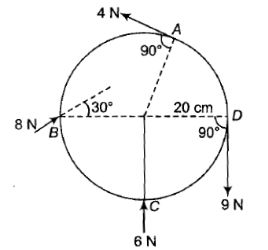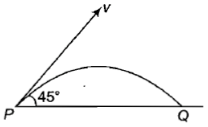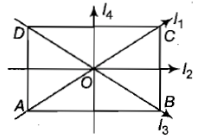A body is rolling without slipping on a horizontal surface and its rotational kinetic energy is equal to the translational kinetic energy. The body is

1.  Disc

2.  Sphere

3.  Cylinder

4.  Ring

Concept Questions :-

Moment of inertia
High Yielding Test Series + Question Bank - NEET 2020

Difficulty Level:

A wheel of radius 20 cm forces applied to it as shown in the figure. The torque produced by the forces 4 N at A, 8 N at B, 6 N at C at D at angles indicated is1.  5.4 N-m anticlockwise

2.  1.80 N-m clockwise

3.  2.0 M-m clockwise

4.  3.6 n-m clockwise

Concept Questions :-

Torque
High Yielding Test Series + Question Bank - NEET 2020

Difficulty Level:

A projectile of mass m is fired with velocity v from a point P, as shown. Neglecting air resistance, the magnitude of the change in momentum between the points P and arriving at Q is1.  Zero

2.  $\frac{\mathrm{mv}}{\sqrt{2}}$

3.

4.  2 mv

Concept Questions :-

Linear momentum
High Yielding Test Series + Question Bank - NEET 2020

Difficulty Level:

The moment of inertia of a thin rectangular plate ABCD of uniform thickness about an axis passing through the centre O and perpendicular to the plane of the plate is1.

2.

3.

4.

Concept Questions :-

Moment of inertia
High Yielding Test Series + Question Bank - NEET 2020

Difficulty Level:

The centre of a wheel rolling on a plane surface moves with a speed ${\mathrm{v}}_{0}$. A particle on the rim of the wheel at the same level as the centre will be moving at speed

1.  zero

2.  ${\mathrm{v}}_{0}$

3.  $\sqrt{2}{\mathrm{v}}_{0}$

4.  $2{\mathrm{v}}_{0}$

Concept Questions :-

Rolling motion
High Yielding Test Series + Question Bank - NEET 2020

Difficulty Level:

A man weighing 80 kg is standing in a trolley weighing 320 kg. The trolley is resting on frictionless horizontal rails. If the man starts walking on the trolley with a speed of 1 m/s, then after 4 sec his displacement relative to the ground will be

(1) 5  m

(2) 4.8 m

(3) 3.2 m

(4) 3.0 m

Concept Questions :-

Center of mass
High Yielding Test Series + Question Bank - NEET 2020

Difficulty Level:

Moment of inertia of a uniform cylinder of mass M, radius R and length l about an axis passing through its center and normal to its axis would be

1.  $\frac{{\mathrm{Ml}}^{2}}{12}$

2.  $\frac{{\mathrm{MR}}^{2}}{2}$

3.

4.

Concept Questions :-

Moment of inertia
High Yielding Test Series + Question Bank - NEET 2020

Difficulty Level:

A particle moves along a circle of radius $\frac{20}{\mathrm{\pi }}m$ with constant tangential acceleration. If the velocity of the particle is 80 m/s at the end of the second revolution after motion has begin, the tangential acceleration is

(1) 640

(2) 160

(3) 40

(4) 40 $\mathrm{m}/{\mathrm{s}}^{2}$

Concept Questions :-

Rotation motion : Introduction
High Yielding Test Series + Question Bank - NEET 2020

Difficulty Level:

If the radius of the earth is suddenly contracted to half of its present value, then the duration of the day will be of

1.  6 hours

2.  12 hours

3.  18 hours

4.  24 hours

Concept Questions :-

Angular momentum
High Yielding Test Series + Question Bank - NEET 2020

Difficulty Level:

A wheel has angular acceleration of 3.0 rad/$se{c}^{2}$ and an initial angular speed of 2.00 rad/sec.  In a time of 2 sec it has rotated through an angle (in radian) of

(1) 10                (2) 12

(3) 4                  (4) 6